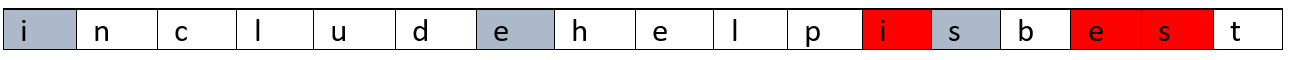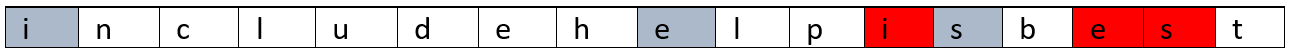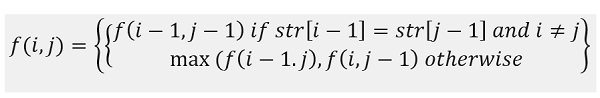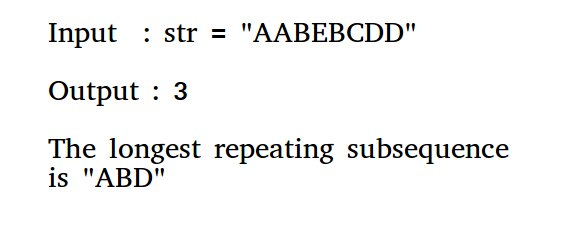• 最长重复子序列Description: 描述： This question has been featured in interview rounds of Amazon. 这个问题已在亚马逊的采访回合中提到。 Problem statement: 问题陈述： Given string str, find the ...
最长不重复子序列Description:
描述：
This question has been featured in interview rounds of Amazon.
这个问题已在亚马逊的采访回合中提到。
Problem statement:
问题陈述：
Given string str, find the length of the longest repeating subsequence such that the two subsequences don't have the same character in the same position, i.e., any ith character in the two sub-sequences shouldn't have the same index in its original string.
给定字符串str ，求出最长重复子序列的长度，以使两个子序列在相同位置没有相同的字符，即，两个子序列中的任何第 i 个字符在其位置均不应具有相同的索引原始字符串。
    Let the string be,
Str="includehelpisbest"

Output will be:
Longest repeating sub-sequence length is 3
The longest repeating sub-sequence is:"ies"The output is given above where the repeating sub-sequences are in two different colours. One more repeating subs-sequence can be,
上面给出了输出，其中重复子序列使用两种不同的颜色。 可以再重复一个子序列，Solution Approach:
解决方法：
The problem can be solved in similar way as longest Common subsequence problem, where input to LCS() would be String str both the case. That is
可以通过与最长公共子序列问题类似的方法来解决该问题 ，在这两种情况下， LCS()的输入均为String。 那是
    LRS(str) = LCS(str,str) which some constraints

Let's discuss the recursive approach first.
让我们首先讨论递归方法。
Let,
让，

l = Length of the  string,str
f(l,l) = Longest repeating subsequence length for string length l

Now,
现在，
Think of the following example,
考虑以下示例，
Say the string is: x1x2...xl
假设字符串是： x 1 x 2 ... x l
Say,
说，
xi==xj and i≠j (this is the constraint what we discussed above)
x i == x j和i≠j (这是我们上面讨论的约束)
Then obviously we need to find LCS for the remaining part of string (x1x2...xi-1, x1x2...xj-1) and then add 1 for this valid character match (repeating character match),
那么显然我们需要为字符串的其余部分(x 1 x 2 ... x i-1 ，x 1 x 2 ... x j-1 )找到LCS，然后为这个有效的字符匹配添加1(重复字符比赛)，
Else
其他
Maximum of two case,
最多两种情况
LCS of the string leaving character xi,x1x2...xi-1 and string x1x2...xj. 字符串x i ，x 1 x 2 ... x i-1和字符串x 1 x 2 ... x j的 LCS。 LCS of the string xl1, x1x2...xi and second string leaving character xj,x1x2...xj-1 字符串x l 1 ，x 1 x 2 ... x i的 LCS和第二个字符串剩下的字符x j ，x 1 x 2 ... x j-1 Now, we need to recur down to 0. So,
现在，我们需要递归降至0。因此，Where base cases are,
在基本情况下，
    f(0,i)=0 for 0≤i≤l
f(i,0)=0 for 0≤i≤ l

If you generate this recursion tree, it will generate many overlapping sub-problems and thus, we need to reduce the re-computing. That's why we need to convert it into dynamic programming where we will store the output of the sub-problems and we will use it to compute bigger sub-problems.
如果生成此递归树，它将生成许多重叠的子问题，因此，我们需要减少重新计算。 这就是为什么我们需要将其转换为动态编程，以便在其中存储子问题的输出，并使用它来计算更大的子问题。
Converting to Dynamic programming:
转换为动态编程：
    1)  Initialize dp[l+1][l+1]  to 0
2)  Convert the base case of recursion:
for i=0 to l
dp[i]=0;
for i=0 to l
dp[i]=0;

3)  Fill the DP table as per recursion.
for i=1 to l    //i be the subproblem length for first string of LCS
for j=1 to l //j be the subproblem length for second string of LCS
if(i≠j and str1[i-1]==str2[j-1]) //xi==xj  and i≠j
dp[i][j]=dp[i-1][j-1]+1;
else
dp[i][j]=max(dp[i-1][j],dp[i][j-1]);
end for
end for
4)  The final output will be dp[l][l]

C++ Implementation:
C ++实现：
#include <bits/stdc++.h>
using namespace std;

void print(vector<int> a, int n)
{
for (int i = 0; i < n; i++)
cout << a[i] << " ";
cout << endl;
}

int max(int a, int b)
{
return (a > b) ? a : b;
}

int LRS(string str1, string str2)
{
int l = str1.length();
int dp[l + 1][l + 1];

//base case
for (int i = 0; i <= l; i++)
dp[i] = 0;

for (int i = 0; i <= l; i++)
dp[i] = 0;

//fill up
for (int i = 1; i <= l; i++) {
for (int j = 1; j <= l; j++) {
if (i != j && str1[i - 1] == str2[j - 1])
dp[i][j] = dp[i - 1][j - 1] + 1;
else
dp[i][j] = max(dp[i - 1][j], dp[i][j - 1]);
}
}

return dp[l][l];
}

int main()
{
string str;

cout << "Enter the string:\n";
cin >> str;
cout << "Longest repeating sub-sequence length: " << LRS(str, str) << endl;

return 0;
}

Output
输出量
Enter the string:
includehelpisbest
Longest repeating sub-sequence length: 3


翻译自: https://www.includehelp.com/icp/longest-repeating-subsequence.aspx最长不重复子序列
展开全文字符串 python 算法 java leetcode
• JAVA算法：最长重复子序列（JAVA） Longest Repeating Subsequence Given a string, find length of the longest repeating subseequence such that the two subsequence don’t have same string character at ...
JAVA算法：最长重复子序列（JAVA）

Longest Repeating Subsequence
Given a string, find length of the longest repeating subseequence such that the two subsequence don’t have same string character at same position, i.e., any i’th character in the two subsequences shouldn’t have the same index in the original string.算法设计

package com.bean.algorithm.basic;

public class LongestRepeatingSubsequence {
// Function to find the longest repeating subsequence
static int findLongestRepeatingSubSeq(String str) {
int n = str.length();

// Create and initialize DP table
int[][] dp = new int[n + 1][n + 1];

// Fill dp table (similar to LCS loops)
for (int i = 1; i <= n; i++) {
for (int j = 1; j <= n; j++) {
// If characters match and indexes are not same
if (str.charAt(i - 1) == str.charAt(j - 1) && i != j)
dp[i][j] = 1 + dp[i - 1][j - 1];

// If characters do not match
else
dp[i][j] = Math.max(dp[i][j - 1], dp[i - 1][j]);
}
}
return dp[n][n];
}

// driver program to check above function
public static void main(String[] args) {
String str = "aabb";
System.out.println("The length of the largest subsequence that" + " repeats itself is : "
+ findLongestRepeatingSubSeq(str));
}
}


程序运行结果：

The length of the largest subsequence that repeats itself is : 2

另外一种算法设计——递归算法 Recursion

package com.bean.algorithm.basic;

import java.util.Arrays;

public class LongestRepeatingSubsequence2 {
static int dp[][] = new int;

// This function mainly returns LCS(str, str)
// with a condition that same characters at
// same index are not considered.
static int findLongestRepeatingSubSeq(char X[], int m, int n) {

if (dp[m][n] != -1) {
return dp[m][n];
}

// return if we have reached the end of either string
if (m == 0 || n == 0) {
return dp[m][n] = 0;
}

// if characters at index m and n matches
// and index is different
if (X[m - 1] == X[n - 1] && m != n) {
return dp[m][n] = findLongestRepeatingSubSeq(X, m - 1, n - 1) + 1;
}

// else if characters at index m and n don't match
return dp[m][n] = Math.max(findLongestRepeatingSubSeq(X, m, n - 1), findLongestRepeatingSubSeq(X, m - 1, n));
}

// Longest Repeated Subsequence Problem
static public void main(String[] args) {
String str = "aabb";
int m = str.length();
for (int[] row : dp) {
Arrays.fill(row, -1);
}
System.out.println("The length of the largest subsequence that" + " repeats itself is : "
+ findLongestRepeatingSubSeq(str.toCharArray(), m, m));

}
}


程序运行结果：

The length of the largest subsequence that repeats itself is : 2


展开全文算法分析与设计 JAVA算法
• 最长重复子序列问题除了使用穷举法，还可以使用后缀数组和后缀树来求解。这里给出使用后缀数组解决的最长重复子序列的过程，并以“banana”为例进行演示。首先写下一个比较两个字符串从头开始共同部分的长度的函数：...
最长重复子序列问题除了使用穷举法，还可以使用后缀数组和后缀树来求解。这里给出使用后缀数组解决的最长重复子序列的过程，并以“banana”为例进行演示。首先写下一个比较两个字符串从头开始共同部分的长度的函数：
int comlen(char *p, char *q) {
int i = 0;
while(*p&&(*p++ == *q++))
i++;
return i;
}
设定该程序最多处理MAXN个字符，并存放在数组c中，a是对应的后缀数组：
#define MAXN 5000000
char c[MAXN],*a[MAXN];
读取输入时，对a进行初始化：
//n是已读入的字符数目
int input(int n) {
int ch;
while((ch = getchar())!=EOF) {
a[n] = &c[n];
c[n++] = ch;
}
c[n] = 0;
return n;
}
这样，对于“banana”，对应的后缀数组为：
a:banana
a:anana
a:nana
a:ana
a:na
a:a
它们是"banana"的所有后缀，这也是“后缀数组”命名原因。
如果某个长字符串在数组c中出现了两次，那么它必然出现在两个不同的后缀中，更准确的说，是两个不同后缀的同一前缀。通过排序可以寻找相同的前缀，排序后的后缀数组为：
a:a
a:ana
a:anana
a:banana
a:na
a:nana
扫描排序后的数组的相邻元素就能得到最长的重复字串，本例为“ana”。
这里做一个扩展：（习题16.8）如何寻找至少出现过n次的最长重复子序列？
解法是比较a[i...i+n]中第一个和最后一个的最长公共前缀长度，上文是对n=1的特例。因此写出一般化的扫描函数：int scan(int n,int k) {
int maxi=0,i;
int temp,maxlen = 0;
for(i=0;i<n-k;i++) {
temp = comlen(a[i],a[i+k]);
if(temp>maxlen) {
maxlen = temp;
maxi = i;
}
}
printf("%d times the longest:%.*s\n",k,maxlen,a[maxi]);
return 0;
}
int pstrcmp(char **p, char **q)
{   return strcmp(*p, *q); }

int main(void) {
int i,n;
n = input(0);
qsort(a,n,sizeof(char *),pstrcmp);
printf("\n");
for(i=0;i<n;i++)
printf("%s\n",a[i]);
scan(n,1);
scan(n,2);
return 0;
}
展开全文• 分析：求最长重复子序列，即说明要找到一个至少出现两次的最长的子序列。假设某个子序列第二次出现和第一次出现的位置相差i，则i的值为1,2，、、、，str.size()-1，代码如下所示： string longestRepeatSubstring...
分析：求最长重复子序列，即说明要找到一个至少出现两次的最长的子序列。假设某个子序列第二次出现和第一次出现的位置相差i，则i的值为1,2，、、、，str.size()-1，代码如下所示：

string longestRepeatSubstring(const string&str)
{
int n = str.size();
if (n==0)
return NULL;
int maxLength = 0;
int startIndex = 0;for (int i = 1; i < n; ++i)
{
int current = 0;
for (int j = 0; j < n-i; ++j)
{
if (str[j] == str[i+j])current++;
else
current = 0;
if (current > maxLength)
{
maxLength = current;
startIndex = j-current+1;
}
}
}
if (maxLength > 0)
{
cout << maxI << endl;
return str.substr(startIndex,maxLength);
}
}

转载于:https://www.cnblogs.com/happygirl-zjj/p/4876882.html
展开全文• 字符串和数组在存储上是类似的，把它们归为同一主题之下。本文主要介绍三大类问题和它们...以字符串散列为例的哈希表最长重复子序列问题的后缀数组解法最大连续子序列 基本问题 直接解法O(n2)：优化解法与累加
• 字符串和数组在存储上是类似的，把它们归为同一主题之下。本文主要介绍三大类问题和它们衍生的问题，以及相应算法。 ... 字符串循环移位（左旋转）问题 ...最长重复子序列问题的后缀数组解法 最大连续子序列 基本...
• 如输入abcdeabcf，则输出abc。 程序如下： /* * author:xincici * brief:输入一个字符串，输出该字符串的最长重复子序列。 */ import java.util.ArrayList; import java.util.List; imstring exception class
• 最长重复子数组 子数组是连续的 类似LCS ，LCS是不要求连续的 递推式： A[i] = B[j]时：memo[i][j] = memo[i-1][j-1] + 1 第一行或第一列，A[i] = B[j]时：memo[i][j] = 1 其他情况 memo[i][j] = 0 LCS 递推式：...
• 题目描述：输入两个整数序列A和B，输出同时在A,B中间出现的最长子序列长度，注意，子序列由原序列的连续元素构成 输入： 第一行第一个数n，表示序列A的长度 第二行n个数，表示序列A 第三行第一个数m，表示序列B...
• 最长重复子序列 https://leetcode-cn.com/problems/longest-increasing-subsequence/ 给你一个整数数组 nums ，找到其中最长严格递增子序列的长度。 子序列是由数组派生而来的序列，删除（或不删除）数组中的元素而...
• 本文为转载。原文地址：... 谢谢作者的智慧与分享！ ... 输入一行字符串，找出其中出现的相同且长度最长的字符串，输出它。   2、解析  例如“yyabcdabjcabceg”，输出应该为abc和3。算法
• 这个深是指从root所经历过的字符个数，最深非叶节点所经历的字符串起来就是最长重复子串。    为什么要非叶节点呢?因为既然是要重复，当然叶节点个数要>=2。    (4). 两个...算法
•  2)重复1)中的操作直到遇到结束符'\0',若当前结点p不为空并且isStr为true，则返回true，否则返回false。 3.删除  删除可以以递归的形式进行删除。 测试程序: /*Trie树(字典树) 2011.10.10*/ ...算法
• 1 #include "stdio.h" 2 #define maxn 20010 3 4 int wa[maxn],wb[maxn],wv[maxn],ws[maxn]; 5 int rank[maxn],height[maxn]; 6 int r[maxn],sa[maxn],ans[maxn]; 7 int n,res,k;... 9 int cmp(...
• 两个题目意思差不多，都是让求最长公共子串，只不过poj那个让输出长度，而URAL那个让输出一个任意的最长的子串。 解体思路： Long Long Message Time Limit: 4000MS   ...
• 现在问其中有最长的相同的子串是多长..首先要没有重合..再一个子串可以是一段全部加/减一个数和另一个子串比较..  题解:  熟悉模板... Program: #include #include #include #include #include #...后缀数组
• 这个深指从root所经历过的字符个数，最深非叶子节点所经历的字符串起来就是最长重复子串。为什么非要是叶子节点呢？因为既然是要重复的，当然叶子节点个数要>=2 4.两个字符串S1,S2的最长公共子串（而非以前所说...算法
• 最长重复子数组 0 1143. 最长公共子序列 给定两个字符串 text1 和 text2，返回这两个字符串的最长公共子序列的长度。 一个字符串的 子序列 是指这样一个新的字符串：它是由原字符串在不改变字符的相对顺序的情况下...
• 文章目录1 最长公共子序列2 最长重复子数组 1 最长公共子序列  定两个字符串 text1 和 text2，返回这两个字符串的最长公共子序列的长度。 一个字符串的 子序列 是指这样一个新的字符串：它是由原字符串在不改变字符...动态规划 leetcode 算法
• 1、最长递增子序列 链接：https://www.nowcoder.com/questionTerminal/585d46a1447b4064b749f08c2ab9ce66 来源：牛客网 对于一个数字序列，请设计一个复杂度为O(nlogn)的算法，返回该序列的最长上升子序列的长度...c++
• 最长公共子序列|最长公共子串|最长重复子串|最长不重复子串|最长回文子串|最长递增子序列|最大子数组和 2012年6月27日Yx.Ac发表评论阅读评论 文章作者：Yx.Ac 文章来源：勇幸|Thinking ...编程语言 c++ 算法
• 最长公共子序列 1、动态规划解决过程 1）描述一个最长公共子序列 　如果序列比较短，可以采用蛮力法枚举出X的所有子序列，然后检查是否是Y的子序列，并记录所发现的最长子序列。如果序列比较长，这种方法需要指数...
• 最长重复子串，最长公共子序列， 最长公共子串原题：首先这是一个单字符串问题。子字符串R 在字符串L 中至少出现两次，则称R 是L 的重复子串。重复子串又分为可重叠重复子串和不可重叠重复子串，这里只是简单讨论...算法 c语言 字符流...